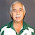## Monday, December 26, 2022

### Selecting Dispersed Points

Fellow blogger Erwin Kalvelagen posted a comparison of two binary programming models, one quadratically constrained and one linearly constrained, for the problem of selecting a maximal number of points from a finite set subject to the requirement that no two selected points be closer than a specified distance. The models were an answer to a question posted on Computational Science Stack Exchange. Not surprisingly, the linearized version tended to solve faster than the quadratic version.

In Erwin's linear model, the constraints take the form $$\underline{D}(x_i + x_j - 1) \le d_{i,j} \quad (1)$$ where $d_{i,j}$ is the distance between points $i$ and $j$, $\underline{D}$ is the minimum allowable distance between selected points, and $x_k$ is a binary variable indicating whether point $k$ is selected (1) or not (0). I coded both his models in Java, using CPLEX 22.1.1, along with another linear model where the constraints are expressed as $$x_i + x_j \le 1\quad (2)$$ for those pairs $(i,j)$ where $d_i + d_j \le \underline{D}.$ In other words, we exploit the fact that we know the distances at the outset to precompute which pairs of points can / cannot coexist, and just rule out the pairs that cannot. Since (1) is equivalent to $$x_i + x_j \le 1 + \frac{d_{i,j}}{\underline{D}},$$ constraint (2) is clearly at least a bit tighter than constraint (1).

Erwin started with a problem size of 50 points for demonstration purposes, then doubled that to compare timing of this two models. I ratcheted the problem size up to 1,000 points to compare his linear model to mine. (I did not test the quadratic model at that size.) As with all things MIP, the results were not entirely consistent. In limited testing, the model using (2) was usually faster than the model using (1), but occasionally (1) proved faster. The run time differences were not large enough to be exciting. For instance, in one test run version (1) needed 4.633 seconds versus 2.987 seconds for version (2).

Overall, I can't say the time differences lived up to my expectations, and the fact that at least occasionally (1) was faster than (2) (perhaps due to some quirk in presolving, or just to some random choices in branching) is consistent with my experience that MIP behaviors are, well, not consistent.

1.Did (1) and (2) yields different presolved models?

1.Yes, with the model using (2) typically smaller. On one particular instance the final presolve report from CPLEX using (1) listed 1238 rows, 684 columns, and 31775 nonzeros, with a clique table containing 1035 members. On the same instance using (2) that became 042 rows, 662 columns, and 26833 nonzeros with 1035 clique table members. (All columns are binary in both.)

2.Lemma: the difference in performance between poor and great formulations has decreased because solvers have become smarter. Better presolvers and better cut generation has led to much better performance for poorly formulated models.

1.I'll accept that as a conjecture (you didn't offer a proof ;-)), with which I agree. I do still encounter formulations that require improvement by the modeler, but I generally operate on the assumption that the solver is smarter than I am, so I don't agonize over "minor" tweaks.

Due to intermittent spamming, comments are being moderated. If this is your first time commenting on the blog, please read the Ground Rules for Comments. In particular, if you want to ask an operations research-related question not relevant to this post, consider asking it on Operations Research Stack Exchange.